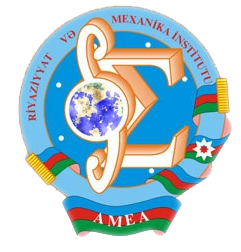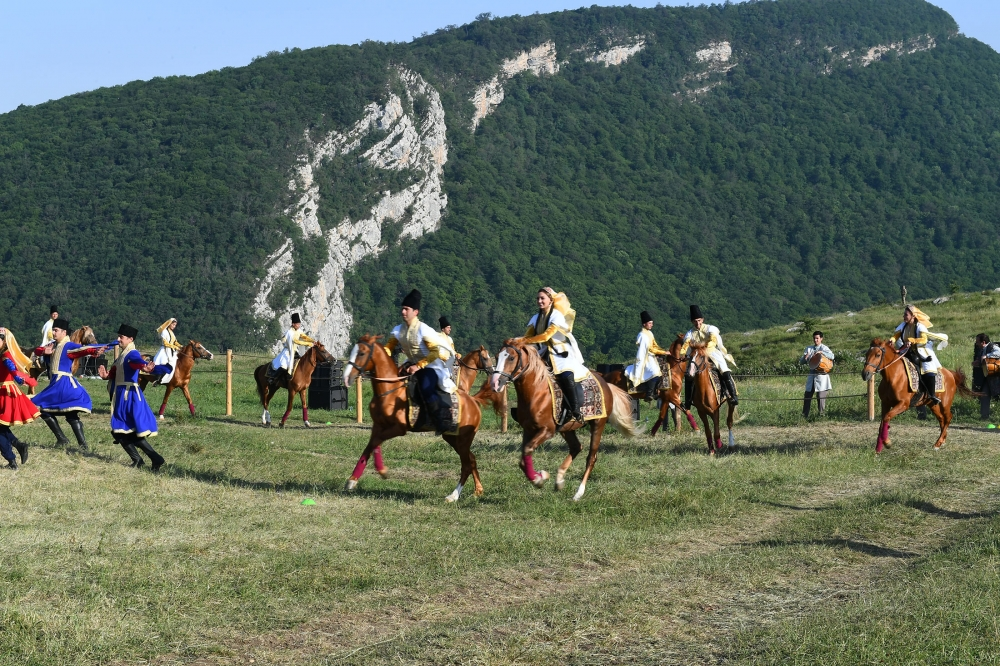Riyaziyyat və Mexanika İnstitutu

## Qarabağ xəbərləri### Şuşada Heydər Əliyev Fondunun təşkilatçılığı ilə “Musiqi irsi və Qarabağ atları Cıdır düzündə” adlı kompozisiya təqdim olunub## Proceedings 2008/29/XXXVII

 Year: 2008 Volume: XXIX Number: XXXVII MATHEMATICS Aliev B.A. Abstract PDF Solvability of boundary value problems for a second order elliptic differential-operator equation with a spectral parameter in both the equation and boundary conditions [PDF] 3 Aslanova N.M. Abstract [PDF] Asymptotic distribution of eigenvalues and regularized trace of boundary value problem for Bessel’s operator equation with eigenvalue dependent boundary condition [PDF] 21 Bilalov B.T., Shykhammedov A.M. Abstract [PDF] On basis properties of systems of functions in Banach spaces [PDF] 43 Dadashova I.B. Abstract [PDF] Dzjadyk-tamrazov type estimations on the curves in a complex plane in the metric Lp [PDF] 55 Guseynov T.S. Abstract [PDF] Basis properties of unitary systems with degenerating coeffcients in L1 and C [PDF] 59 Huseynov A.H. Abstract [PDF] Existence and uniqueness results for a nonlinear boundary value problem with impulse [PDF] 65 Khudaverdiyev K.I., Heydarova M.N. Abstract [PDF] On existence in large for almost everywhere solution of one-dimensional mixed problem for fourth order semilinear biparabolic equation [PDF] 79 Kuliyev H.F., Jabbarova K.Sh. Abstract [PDF] Application of the l-problem of moments to the solution of optimal control problem for string vibrations equation [PDF] 97 Mamedova V.A. Abstract [PDF] On removable sets of solutions of the second order elliptico-parabolic equations of the second order [PDF] 107 Mamedov F.V. Abstract [PDF] Nonexistence of global solutions for some evolution inequalities of second order [PDF] 117 Mirheydarli M.M. Abstract [PDF] On solvability of Neumann problem for semi-linear elliptic equations with discontinuous coeffcients [PDF] 123 Mirzoev S.S., Mamedov A.M. Abstract [PDF] On an initial boundary-value problem for operator-differential equations of third order in Hilbert space [PDF] 129 Rasulov R.A. Abstract [PDF] Behavior of solutions of nonlinear evolution equations in unbounded domains [PDF] 137 Sadikhov M.N. Abstract [PDF] On existence in large for almost everywhere solution of one-dimensional mixed problem for a class of Korteweg-de Vries-Burgers type nonlinear equations [PDF] 149 Salimova G.A. Abstract [PDF] Inverse boundary value problem for a fourth order partial differential equation with not self-adjoint boundary conditions [PDF] 159 Seidova K.I. Abstract [PDF] Solvability of the second boundary value problem for time dependent Schr¨odinger equation with square summable potential [PDF] 171 Sheﬁyeva G.Kh. Abstract [PDF] On determination of an unknown coeffcient at higher derivative in a second order parabolic equation [PDF] 177 MECHANICS Aliyev A.B., Ramazanov T.K. Abstract [PDF] On inﬂuence of wall layer of sediment on the motion of suspension in pipe [PDF] 187 Gasanova L.A. Abstract [PDF] Thermo-elastic failure of damaged spherical Vessel [PDF] 193 Kubishev I.N. Abstract [PDF] Bearing capacity of a composite annular plate with different ﬁxing conditions situated the action of non uniformly distributed load [PDF] 199 Latifov F.S., Aliyev F.F. Abstract [PDF] Free axisymmetric vibrations of ﬂowing ﬂuid ribbed cylindrical shell contacting with elastic medium [PDF] 209 Shahbandaev E.G. Abstract [PDF] On partial closing of cracks in heat-releasing medium weakened by a periodic system of circular holes [PDF] 215 APPLIED PROBLEMS OF MATHEMATICS AND MECHANICS Jabrailov Sh.I. Abstract [PDF] Optimality condition for control of systems with three-point boundary conditions [PDF] 225 Mahmudov N.M. Abstract [PDF] An optimal control problem for Schrodinger’s equation with real-valued coeffcient in the non-linear part of the equation [PDF] 231 Salmanov V.J. Abstract [PDF] Pontryagin’s maximum principle in an optimal control problem for ordinary differential equations with special quality test [PDF] 239 Tagiyev R.K. Abstract [PDF] On optimal control for quasi-linear elliptic equations [PDF] 247
Azərbaycanda COVID-19 ilə bağlı statistika
• Virusa yoluxan

596973

• Sağalan

565521

• Yeni yoluxan

0

• Aktiv xəstə

23469

• Ölüm halı

7983

• Test edilib

5,608,158